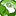# QlikView App Dev

Discussion Board for collaboration related to QlikView App Development.

Announcements
Action-Packed Learning Awaits! QlikWorld 2023. April 17 - 20 in Las Vegas: REGISTER NOW
cancel
Showing results for
Did you mean:Creator

## Replacing only character in a specific location on the string via qv load

hello

I need to replace it with an M if D is position one..

for this one I got the if statement but the replace is replacing all the D in the code.. I just want the first one.. and im not sure why its not working below.

if(left(code,1)='D',replace(code,(left(code,1)),'M')) as code2

or delete the D if its in the 2nd position and code length greater that 11.

not sure how to do part 2..

if(left(code,2)='D',(if(count,code)=11)

thanks

1 Solution

Accepted SolutionsMVP

May be this for Part 1:

If(Left(code, 1) = 'D', 'M' & Right(code, (Len(code) - 1)))

7 RepliesMVP

May be this for Part 1:

If(Left(code, 1) = 'D', 'M' & Right(code, (Len(code) - 1)))MVP

May be this for Part 2:

If(Mid(code, 2, 1) = 'D' and Len(code) = 11, Left(code, 1) & Right(code, 9))Creator
Author

had to change the above to length to 12 but it works..

do you know how I can put this in one if statement?

right now when I do this.. It makes 2 columns  of data..

if code 1  = column data 1

if code 2 = column data 2

how do I run both to get it into one column data 1

thanksMVP

I guess which one do you want to check first? First Letter = 'D' or the Second Letter = 'D'?

If First Letter = 'D', then try this:

If(Left(code, 1) = 'D', 'M' & Right(code, (Len(code) - 1)),

If(Mid(code, 2, 1) = 'D' and Len(code) = 11, Left(code, 1) & Right(code, 9)

, ElseConditionIfNeeded

))

If Second Letter = 'D'

If(Mid(code, 2, 1) = 'D' and Len(code) = 11, Left(code, 1) & Right(code, 9)

If(Left(code, 1) = 'D', 'M' & Right(code, (Len(code) - 1))

, ElseConditionIfNeeded

))Creator
Author

hi sunny

everytime I do

If(Left(code, 1) = 'D', 'M' & Right(code, (Len(code) - 1)),

If(Mid(code, 2, 1) = 'D' and Len(code) = 11, Left(code, 1) & Right(code, 9) as code2)),

I get an missing ) error...MVP

You probably need this:

If(Left(code, 1) = 'D', 'M' & Right(code, (Len(code) - 1)),

If(Mid(code, 2, 1) = 'D' and Len(code) = 11, Left(code, 1) & Right(code, 9))) as code2,Creator
Author

perfect

thank you so muchCommunity Browser